# Particle Systems

by Allen Martin

## Introduction

The term particle system is loosely defined in computer graphics. It has been used to describe modeling techniques, rendering techniques, and even types of animation. In fact, the definition of a particle system seems to depend on the application that it is being used for. The criteria that hold true for all particle systems are the following: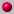Collection of particles - A particle system is composed of one or more individual particles. Each of these particles has attributes that directly or indirectly effect the behavior of the particle or ultimately how and where the particle is rendered. Often, particles are graphical primitives such as points or lines, but they are not limited to this. Particle systems have also been used to represent complex group dynamics such as flocking birds.Stochastically defined attributes - The other common characteristic of all particle systems is the introduction of some type of random element. This random element can be used to control the particle attributes such as position, velocity and color. Usually the random element is controlled by some type of predefined stochastic limits, such as bounds, variance, or type of distribution.

This summary will present three different particle systems, two of which were created by Reeves [REE83] [REE85] and one created by Reynolds [REY87]. In addition, images from some example particle systems based on Reeves initial model [REE83] will be presented.

## Using Particle Systems to Model Fire and Explosions

Reeves initially used the term "particle system" to describe a method he used to create a sequence of images for the move Star Trek II: The Wrath of Khan. The effect he was trying to create was that of a bomb exploding on the surface of a planet and fire spreading out from the point of impact to eventually engulf the planet. Each particle in this system was a single point in space. The fire was represented by thousands of these individual points. Typical polygonal rendering methods create objects with straight edges, but representing the fire by thousands of points gives the fire a "fuzzy" shape. Reeves calls an object made up of particles a fuzzy object.

Each object in Reeves particle system had the following attributes:PositionVelocity (speed and direction)ColorLifetimeAgeShapeSizeTransparency

### Particle Life Cycle

Each particle goes through three distinct phases in the particle system: generation, dynamics, and death. These phases are described in more detail here:Generation - Particles in the system are generated randomly within a predetermined location of the fuzzy object. This space is termed the generation shape of the fuzzy object, and this generation shape may change over time. Each of the above mentioned attribute is given an initial value. These initial values may be fixed or may be determined by a stochastic process.Particle Dynamics - The attributes of each of the particles may vary over time. For example, the color of a particle in an explosion may get darker as it gets further from the center of the explosion, indicating that it is cooling off. In general, each of the particle attributes can be specified by a parametric equation with time as the parameter. Particle attributes can be functions of both time and other particle attributes. For example, particle position is going to be dependent on previous particle position and velocity as well as time.Extinction - Each particle has two attributes dealing with length of existence: age and lifetime. Age is the time that the particle has been alive (measured in frames), this value is always initialized to 0 when the particle is created. Lifetime is the maximum amount of time that the particle can live (measured in frames). When the particle age matches it's lifetime it is destroyed. In addition there may be other criteria for terminating a particle prematurely:Running out of bounds - If a particle moves out of the viewing area and will not reenter it, then there is no reason to keep the particle active.Hitting the ground - It may be assumed that particles that run into the ground burn out and can no longer be seen.Some attribute reaches a threshold - For example, if the particle color is so close to black that it will not contribute any color to the final image, then it can be safely destroyed.

### Rendering

When rendering this system of thousands of particles, some assumptions have to be made to simplify the process. First, each particle is rendered to a small graphical primitive (blob). Particles that map to the same pixels in the image are additive - the color of a pixel is simply the sum of the color values of all the particles that map to it. Because of this assumption, no hidden surface algorithms are needed to render the image, the particles are simply rendered in order. Effects like temporal ant-aliasing (motion blur) are made simple by the particle system process. The position and velocity are known for each particle. By rendering a particle as a streak, motion blur can be achieved.

## Using Particle Systems to Model Trees

Reeves used the particle system approach to model trees in the animated short movie The Adventures of Andre and Wally B. [REE85] Each tree was created by using a particle system, and the position of the trees within the forest was also controlled by a particle system. The major enhancement over the previously mentioned particle system is the rendering algorithm used. In The Adventures of Andre and Wally B. a more traditional rendering method is used, and the previous simplification of rendering the particles in order and making them additive is not used. In addition, the generation shape of the particle system is not fixed, but is dependent on the current state. For example, leaves may only be created near the ends of branches.

### Tree Particle System Rendering Technique

The particles of the trees are small circles used to represent leaves and lines used to represent branches. A more traditional rendering technique is used that is based on the painter's algorithm. Because of the large number of particles, standard lighting and shadow calculations are computationally prohibitive, so more reasonable solutions must be made.Self Shadowing - Particles on the outside of the tree are more likely to be directly illuminated then points on the inside of the tree due to the self shadowing of the tree. To model this, a lighting model is used where a cone is used as the bounding volume for the tree. The distance from the point to the outside of the bounding volume along the line between the point and the light source is used as a parameter to the lighting equation. The lighting equation has a maximum value at the outer edge of the bounding volume facing the light, and decreases exponentially inside the tree. The previously mentioned distance parameter is used as the exponent in this equation.Ambient Lighting - Ambient lighting is based on an equation similar to the self shadowing equation. Since ambient light is not based on direct illumination from a light source the distance metric is made from the point being illuminated horizontally out to the edge of the bounding volume.External Shadowing - In addition to shadows cast by the tree on itself, shadows cast from neighboring trees must be considered. This is accomplished by tracing a line from the top of each neighboring tree to the light source. When a particle is being rendered it is checked to see if it is above or below this line. Points above this line are not shadowed, and points below this line have a chance of being in shadow that increases in proportion to the distance from the line.

For the same reasons that shadows could not be perfectly generated for particles within the trees, the shadows that the trees cast on the ground must also be approximated. The way this was done was by rendering an image of the trees from a viewpoint on the ground. This image was then texture mapped to the ground as a "shadow map." Points in the image that correspond to trees were rendered as though they were in shadow.

The actual rendering algorithm used to draw the trees was a modified painter's algorithm. The first assumption used by this algorithm is that the bounding volumes of no two trees intersect, so each tree could be rendered independently. This cuts down on the number of particles that have to be loaded simultaneously. In addition, instead of simply depth sorting the particles, they are placed in a number of depth sorted bins. Inside each bin the particles are simply rendered in order. This saves time because an exhaustive sort of all particles does not have to be made. Errors caused by rendering particles in order inside the bin are masked by the large number of particles.

## Using Particle Systems to Model Flocking Birds

The third example of using particle systems was used by Reynolds to model the behavior of birds moving in a flock [REY87]. In this particle system the particles are used to represent "boids" (short for bird-object). This use of a particle system has a few differences from what was used by Reeves:Each boid is an entire polygonal object rather than a graphical primitiveEach boid has a local coordinate system.There are a fixed number of boids - they are not created or destroyed.Traditional rendering methods can be used because there are a small number of boids.Boids behavior is dependent on external as well as internal state. In other words, a boid reacts to what other boids are doing around it.

### Boid Behavior Model

Reynolds used observation of real flocks and research of flock behavior to come up with three primary needs of a boid.Collision avoidance - The boid does not wish to collide with other boids or obstacles.Velocity matching - Each boid attempts to go the same speed and direction as neighboring boids.Flock centering - Each boid attempts to stay close to nearby flockmates.
The boid's movement is made by combining the impulses that are generated from these three needs. If each need produces a vector that represents the direction and speed it thinks the boid should move in, it may not be adequate to simply average these vectors. In the worst case, these three vectors may be pointing in completely divergent directions, and the net movement would be zero.## Examples

Here are a few particle system examples that I put together to demonstrate the process. These examples demonstrate the algorithm presented by Reeves in [REE83]. Each of the particles maps to a single pixel in the output image. The particles are translucent, with the color of multiple particles that map to the same pixel being additive. In each example the particles are given an initial upwards trajectory and are acted upon by gravity to give an overall parabolic path. Except for the example where there are multiple generation points each of these sequences was created using 20,000 particles and is rendered to a 256x256 MPEG animation. The number of frames in each animation varies from 20 to 50.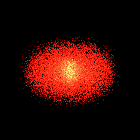Example 1 - Particles are laid out in a circular disc structure and given an initial upwards velocity. When the particle's lifetime is expired it is removed. If the particle collides with the ground it remains stationary.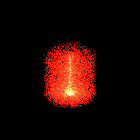Example 2 - Similar to Example 1, but particles are initially clustered near a single point.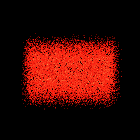Example 3 - Similar to Example 1, but particles are initially laid out in a square structure.Example 4 - Similar to Example 1, but particles are initially laid out in a ring structure.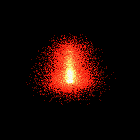Example 5 - In this example the particles are initially clustered in a point similar to Example 2, but particles are constantly being regenerated at the start point. The effect looks very much like a fire or torch burning.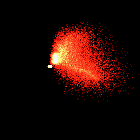Example 6 - In this example the point where the particles are being regenerated is translated in a circular motion through time. The effect looks like a wick burning. Note a visual anomaly with this sequence: there is a small gap between the point where the particles are being generated and where the cluster of generated particles from the previous frame are located. This exists because the initial upwards velocity has a fixed positive lower bound. Particles will have a minimum upwards movement dependent on this velocity in the first frame after they are created.Example 7 - In this example there are multiple generation points. The generation points are randomly created within a square boundary on the ground at random times during the sequence. The particles are created in a cluster at each generation point and are not regenerated. The result looks like a series of volcanos erupting or bombs exploding.## References

[REE83]
W. T. Reeves, "Particle Systems - A Technique for Modeling a Class of Fuzzy Objects", Computer Graphics, vol. 17, no. 3, pp 359-376, 1983.
[REE85]
W. T. Reeves, "Approximate and Probabilistic Algorithms for Shading and Rendering Structured Particle Systems", Computer Graphics, vol. 19, no. 3, pp 313-322, 1985.
[REY87]
C. W. Reynolds, "Flocks, Herds, and Schools: A Distributed Behavioral Model", Computer Graphics, vol. 21, no. 4, pp 25-34, 1987.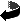Allen Martin / amartin@cs.wpi.edu